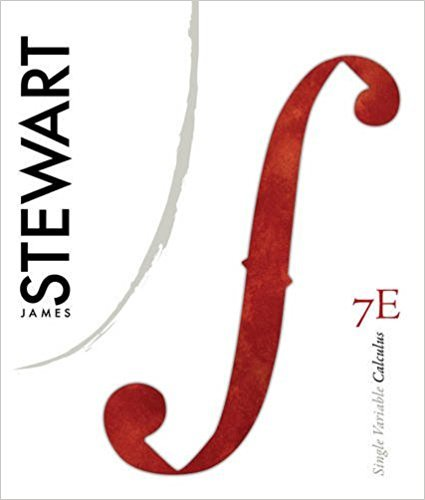×
Get Full Access to Single Variable Calculus - 7 Edition - Chapter 11 - Problem 58
Get Full Access to Single Variable Calculus - 7 Edition - Chapter 11 - Problem 58

×

# Solved: Approximate by a Taylor polynomial with degree atISBN: 9780538497831 151

## Solution for problem 58 Chapter 11

Single Variable Calculus | 7th Edition

• Textbook Solutions
• 2901 Step-by-step solutions solved by professors and subject experts
• Get 24/7 help from StudySoup virtual teaching assistantsSingle Variable Calculus | 7th Edition

4 5 1 373 Reviews
19
3
Problem 58

Approximate by a Taylor polynomial with degree at the number . ; (b) Graph and on a common screen. (c) Use Taylors Inequality to estimate the accuracy of the approximation when lies in the given interval

Step-by-Step Solution:
Step 1 of 3

Step 2 of 3

Step 3 of 3

##### ISBN: 9780538497831

Unlock Textbook Solution